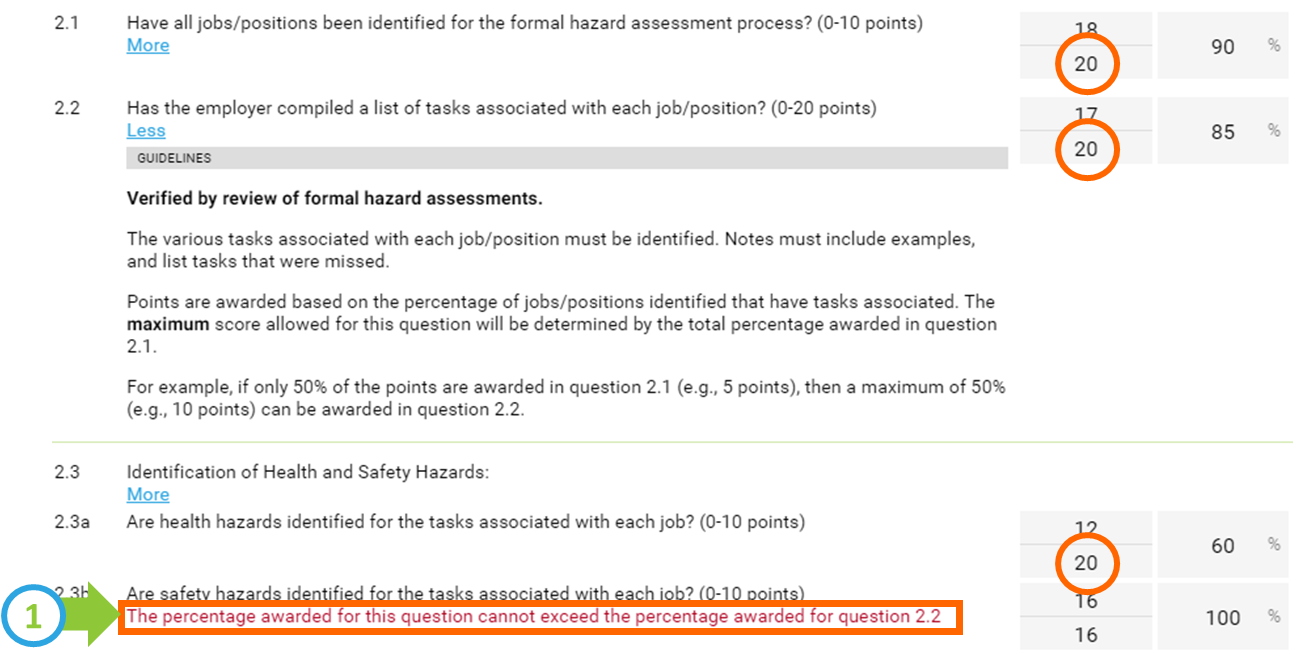The response to some questions in an audit may be restricted based on the response to a prior questions. These question dependencies are built often built into into AuditSoft.  There are two methods that can be used to represent question dependencies:

# Absolute Dependency

In an Absolute dependency scoring model the user has to enforce the dependency rule and AuditSoft will display a warning (see 1 below) when the dependency requirement is violated.

In the example below the base question, in this case 2.1, represents the sample that all dependent questions have to be scored against. All questions are scored based on the absolute number of jobs. For example

• Question 2.1 asks what % of jobs, have been identified for the formal hazard assessment process - the answer is 18 of 20 (90%)
• Question 2.2 determines the % of all jobs (again 20) that have tasks associated, but the answer has to be less than or equal to the number of jobs that have been identified in 2.1 - in this case the answer is 17 of 20 or 85%
• This pattern is continued where the maximum % assigned for each subsequent dependent question must be less than or equal to the previous response. Meaning all questions are answered based on the absolute number of jobs (in this case 20)# Relative Dependency

Relative dependency refers to the scoring of one question relative to another. This is the most common implementation of dependencies. In this scenario the system ensures that the score of one question cannot exceed the score of another question.

In the example below each question is scored based on the available evidence that was reviewed for the question and the system applies a calculation to convert the relative result into and absolute result. For example:

• Question 2.1 asks what % of jobs, have been identified for the formal hazard assessment process - the answer is 18 of 20 (90%) - this is the SAME as the absolute result listed above
• Question 2.2 determines the % of available jobs (in this case 18) that have tasks associated - in this case a relative result or 17 of 18 (94%) is recorded. Through calculation this results in 85% (94% x 90%)  - which is identical to the absolute result of 85% above.
• This pattern is continued where the % assigned for each subsequent question is based on (relative to) the available evidence and is converted by calculation into the appropriate absolute %.